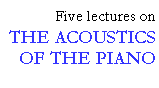### Influence of ribbing

```
```

The purpose of the soundboard is to radiate a large volume of sound over a wide frequency range. This would imply that as much as possible of the energy of the string vibrations should be transferred to the soundboard. However, this is not the whole story. If the transfer of energy is too efficient, the decay of the tone will be too abrupt. In order to achieve a reasonable sustain, the soundboard must reflect a large portion of the vibration energy back to the strings. Soundboard design is thus a compromise.

A stiffening of the soundboard would improve the sound radiation efficiency, as a stiff soundboard is less inclined to subdivide into small vibrating areas. One purpose of the ribs is precisely to stiffen the soundboard, which indeed is thin in proportion to its size. Another purpose is to "homogenize" the soundboard by equalizing the difference in bending stiffness (elasticity modulus) parallel to and across the grain. The moduli of elasticity in the two directions are in a ratio of approximately 20:1 (anisotropism). If this anisotropism is not compensated for by the addition of ribs running across the grain, the effective vibrating area is reduced and the radiation efficiency is decreased over a broad frequency band.

```
```

### Rib height

The ribs should increase the bending stiffness, but not, on the other hand, load the soundboard with too much extra mass. For this reason, experiments were made to determine the general influence of ribs on input impedance and sound radiation. The experiments were conducted on a soundboard for which the height of the ribs was reduced in steps of 25% of the original height. The ribs were planed down using an electrical router, without removing them from the soundboard.

Theoretically the moment of inertia of the ribs determines their stiffness. The moment of inertia (I) of a rib, the cross section of which is assumed to be rectangular, is: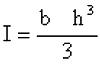where b is the width and h is the height of the cross section. If the height is reduced without changing the width, the result is a rapid decrease in the moment of inertia and hence in the stiffening effect of the rib, while the mass decreases much slower (see Fig. 8)

```
```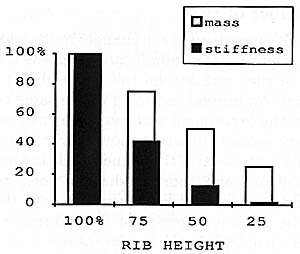Fig. 8. Changes in mass and stiffness of a rib of rectangular cross section as rib height is reduced.

```
```

The experiments showed that at the upper end of the treble bridge, no noticeable change in the input impedance occurred when the rib height was reduced. Here, the ribs do not play a decisive role, either in stiffening or in adding mass. Instead, the boundary conditions at the joint between the soundboard and the frame exert a greater influence.

In the central region of the soundboard, on the other hand, we may observe the development of pronounced resonances (valleys) in the impedance curve below 200 Hz when the rib height is reduced (see Fig. 9). The resonance frequencies are gradually shifted downwards with decreasing rib height. At the same time, the resonances become more sharply defined. The difference in level between peaks and valleys increases, while the average value for the impedance decreases below 200 Hz. Above 500 Hz, the impedance curve is only slightly influenced, and no shift in the upper resonant frequencies can be observed.

```
```

Fig. 9. Influence of ribbing on the input impedance (MP 7):
(a) Original rib height (100 %)
(b) 75 % of original
(c) 50 % of original
(d) 25 % of original and
(e) no ribbing.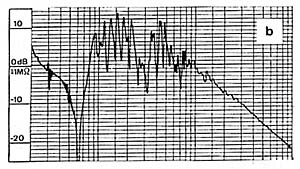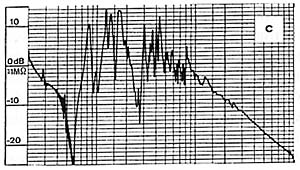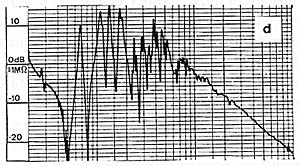```
```

No uniform relation was found between the successive stages of reduction of the rib height and the changes in the input impedance. The influence of the first reduction (from 100 to 75% rib height) was considerably less than that of the second (from 75 to 50%). The influence seems to be greater once the basic stiffening has been removed and there is no longer any compensation for the anisotropism in the moduli of elasticity.

Turning to the sound radiation, the same effects were observed as for the impedance; the lower resonant frequencies are shifted downwards and sound radiation is reduced. However, unlike the effect on the impedance, the reduction in sound radiation extends over the entire frequency range, even above 500 Hz. This is due to a short-circuiting phenomenon, as mentioned in the introduction. Above a certain frequency, the soundboard no longer vibrates as a unit but divides into a number of vibrating areas, which results in a poorer radiation efficiency. In addition, the lack of compensation for the anisotropism causes a reduction in the total vibrating area and hence a poorer radiation.

From the measurements it can be concluded that the two most important functions of the ribs are to stiffen the soundboard and to compensate for the differences in bending stiffness parallel to and across the grain (anisotropism). The bending stiffness exerts a greater influence than the mass. For this reason it is more advantageous to use narrow, high ribs than ribs with a low and wide section.

```
```

### Number of ribs

We also investigated the changes in the acoustic properties of a soundboard when the number of ribs, rather than the rib height, is reduced. An upright assembly of the same type as in the experiment with rib height was used. Every second rib was removed by routing it down to the level of the soundboard, and input impedance and sound radiation were measured. A comparison of these data with those of the previous experiment showed that a reduction of 50% in the number of ribs is equivalent to a 25% reduction in the rib height. This applied both to the input impedance and the sound radiation. The measurements clearly show that a change in the number of ribs exerts less influence on the acoustic properties of a soundboard than a change in rib height, all other parameters being equal.

This lecture is one of Five lectures on the Acoustics of the piano